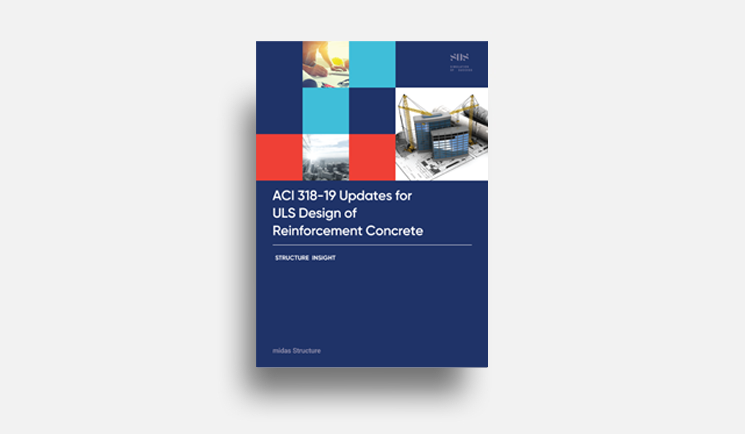# midas Structure

BLOG PROJECT TUTORIAL

# Introduce for the Stability Design of steel structure

Most of the design codes consider all of the following regarding the stability of steel structures and their elements.

(1) Deflection of flexural, shear, and axial members & all other deformations affecting the displacement of the structure

(2) Secondary effect (P-Δ, P-δ)

(3) Geometric imperfections

(4) Stiffness decrease due to inelasticity

(5) Uncertainty of stiffness and strength

The method to design the stability of the structure by reflecting the above requirements is as follows.

- Direct Analysis Method (DA)

- Effective Length Method (ELM)

- Primary Analysis Method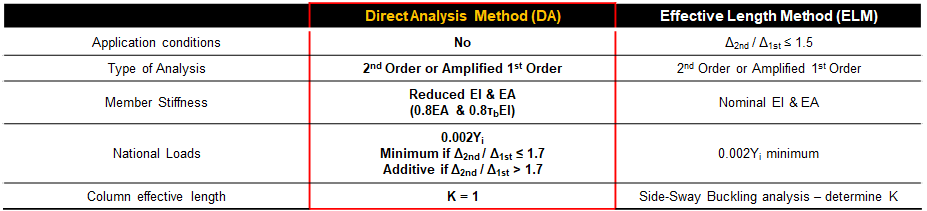Figure 1. Comparison with Direct Analysis Method(DA) & Effective Length Method (ELM)

# Considerations in Direct Analysis

The direct analysis is a newly developed stability design method that recognizes the limitations of ELM in the design of increasingly complex steel structures and is an analysis method that can be used regardless of the structure type.

IIn 2nd analysis, the virtual minimum lateral load, Ni should be included in all gravity load combinations and applied to each floor of the structure.
This is to reflect the initial deformation by assuming that the verticality error during construction is L/500(0.002*L).
.There is also a method of directly modeling the initial deformation instead of applying a virtual lateral load.

IIn case of Δ2nd1st ≤ 1.5, the virtual lateral load is applied only to the gravity load combinations. If this is exceeded, a virtual lateral load is to be added to all load combinations.

IIt is analyzed by reducing the stiffness of all members considering the stiffness uncertainty.
In the case of flexural stiffness, τb factor is additionally applied to consider the stiffness reduction effect due to residual stress.

- EA = 0.8*EA (for axial stiffness)
- EI = 0.8*τb*EI (for flexural stiffness)

Because the direct analysis method reflects the structural uncertainty that affects stability in the analysis model,
and the effective buckling length coefficient, K=1.0 can be used for all members.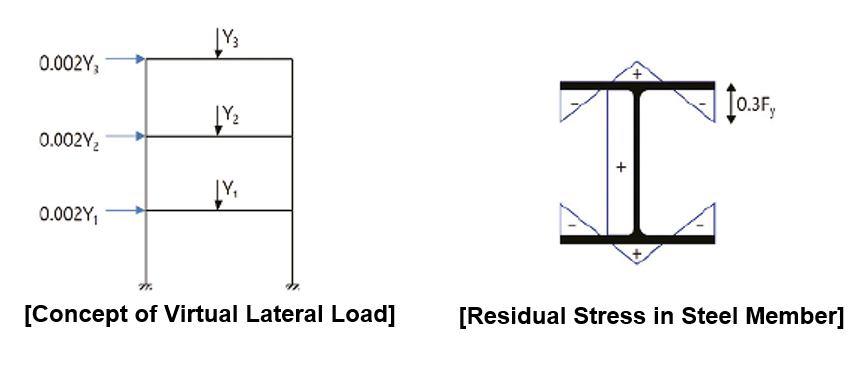Figure 2. Concepts for application of direct analysis

# What is a secondary effect?

When displacement occurs in the structure, additional member force is applied, and the process in which displacement due to additional member force occurs again is repeated. This is called the secondary effect or P-delta effect.
The effect caused by the displacement, δ occurring inside the member is usually called P-δ, and the one caused by the lateral displacement of the frame, Δ is called the P-Δ effect.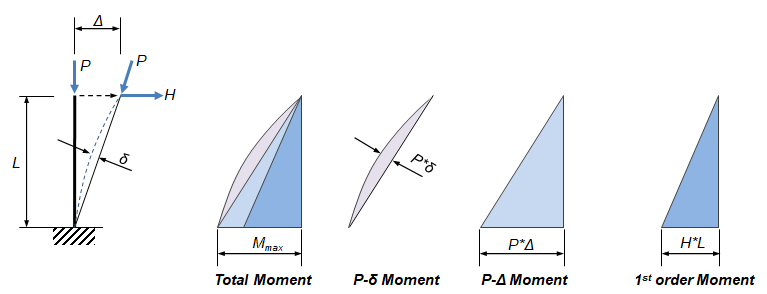Figure 3. P-δ, P-Δ effects of cantilever columns

I2nd analysis means to repeat the re-analysis after modifying the stiffness system until the displacement due to the secondary effect converges to a microscale displacement below a certain level.

The stiffness of the converged structure shows less lateral stiffness than the initial structure, which is a natural result considering that there is an increase in lateral displacement due to the secondary effect.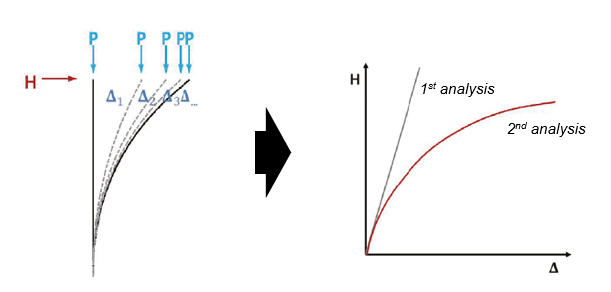Figure 4. Comparision with 1st Analysis & 2nd Anlysis

Looking at the design code(AISC) in relation to the 2nd-order analysis in DA and ELM, it is specified that any 2nd-order analysis method that can consider both P-δ and P-Δ can be used .

According to the characteristics of the structure, there are 3 ways for using 2nd order analysis methods.

Method 1 : Approximate 2nd-order analysis using coefficients B1 and B2,

Method 2 : P-δ effect is considered by applying the B1 coefficient, and the P-Δ is considered through 2nd-order analysis,

Method 3 : Directly considers P-δ and P-Δ by performing a geometric nonlinear analysis.

The approximate 2nd-order analysis is a method of amplifying the member force calculated through 1st-order analysis by applying the coefficients B1 and B2 to consider the P-δ and P-Δ effects, respectively. However, since the B2 coefficient calculation process requires a clear floor division, there is no other way to calculate it if there is no floor division. Therefore, the approximate 2nd-order analysis is a formal and suitable method only for buildings with orthogonal frames, and it is not easy to apply to irregular structures.

The general direct analysis method means the method according to method 2~3.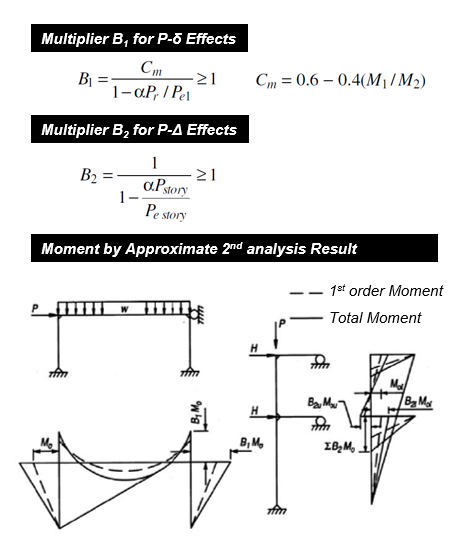Figure 5.Formula in design code (AISC) in relation to the 2nd-order analysis

# The usefulness of Direct Analysis Method

Why use the direct analysis method?

I a) It is the primary method in steel structure design.
I b) It is possible to apply to all types of structural systems.
I c) It can capture the internal structure forces more accurately.
i d) It is the correct design of beams and connections providing rotational column restraint.
e) No need to calculate K-factors.
I f) It is possible to apply for all side-sway amplification values (Δ2nd order / Δ1st order).
g) Effective length method is limited (ELM should be used when Δ2nd order / Δ1st order < 1.5.).

# The usefulness of Direct Analysis Method

## How to  create the imperfection load in MidasGen

The procedure of creating imperfection loads in Midas Gen is as follows.

1) Step 1. Create a model of the lateral force resisting frame, including the leaning column.
2) Step 2. Reduce the stiffness of the lateral framing members in your model.
3) Step 3. Apply notional load or directly model the imperfections.
4) Step 4. Conduct a second-order analysis (“Rigorous” or B1-B2 amplification on first-order).
* Mesh compression elements to capture P-δ.
* “Rigorous” means to use geometric nonlinear analysis.
5) Step 5. Design members using AISC specification and K=1.0.
6) Step 6. Check lateral drift limits for wind and seismic under a unreduced stiffness.

1) Step 1. Create a model of the lateral force resisting frame, including the leaning column.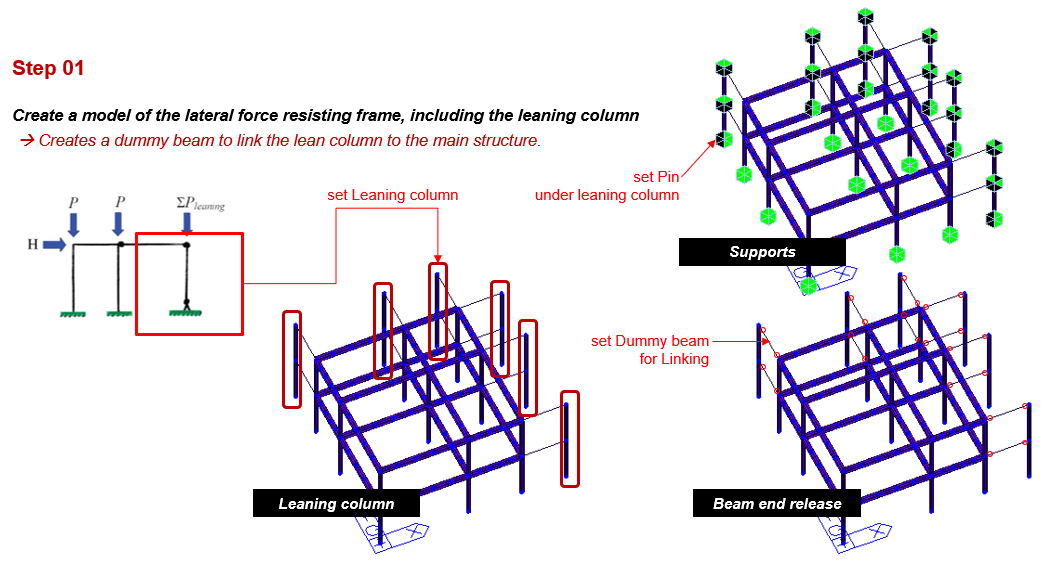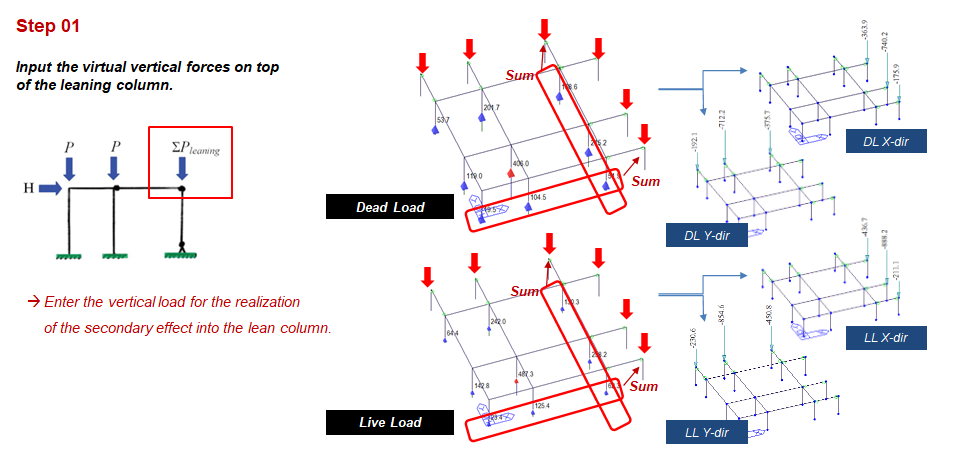Figure 6. The design procedure of direct analysis in Midas Gen (Step 1)

2) Step 2. Reduce the stiffness of the lateral framing members in your model.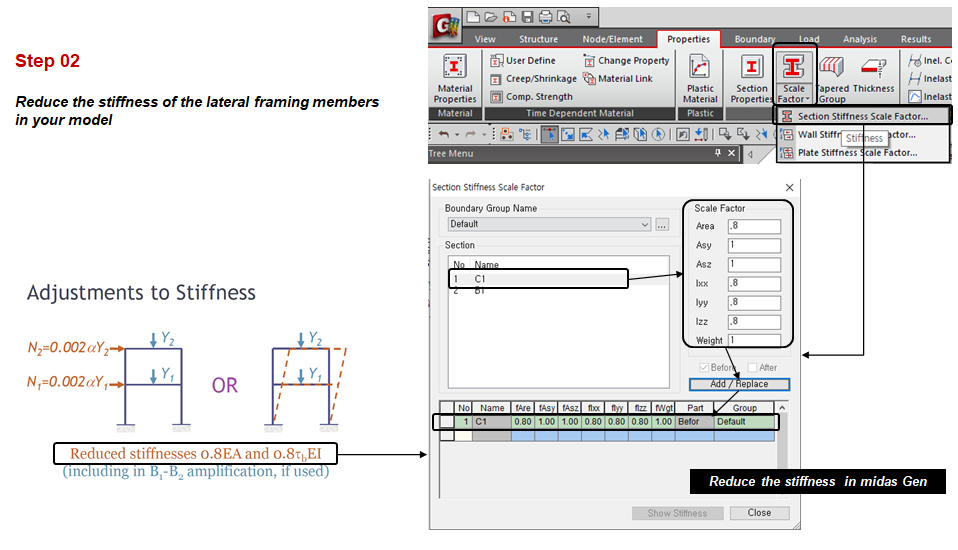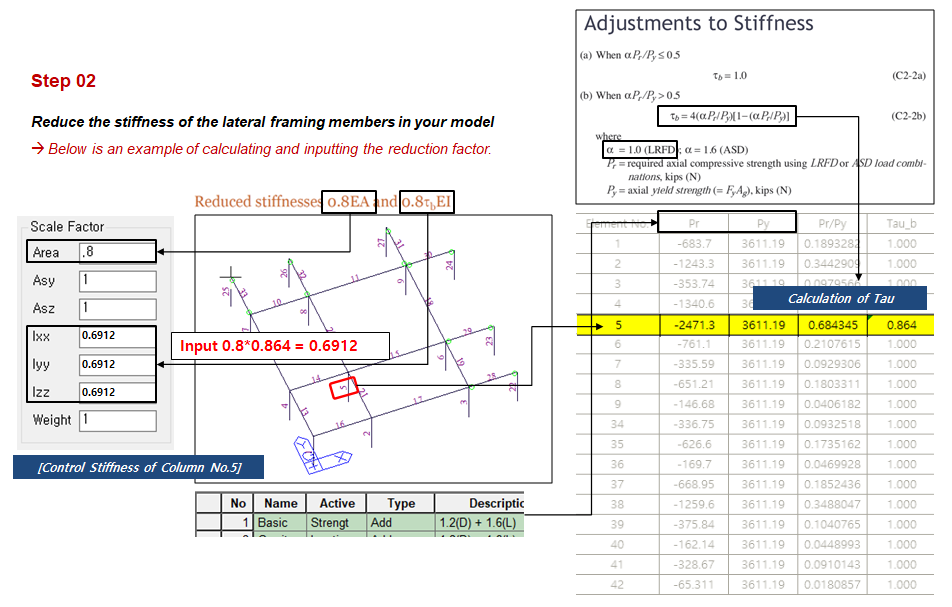Figure 7. The design procedure of direct analysis in Midas Gen (Step 2)

3) Step 3. Apply notional load or directly model the imperfections.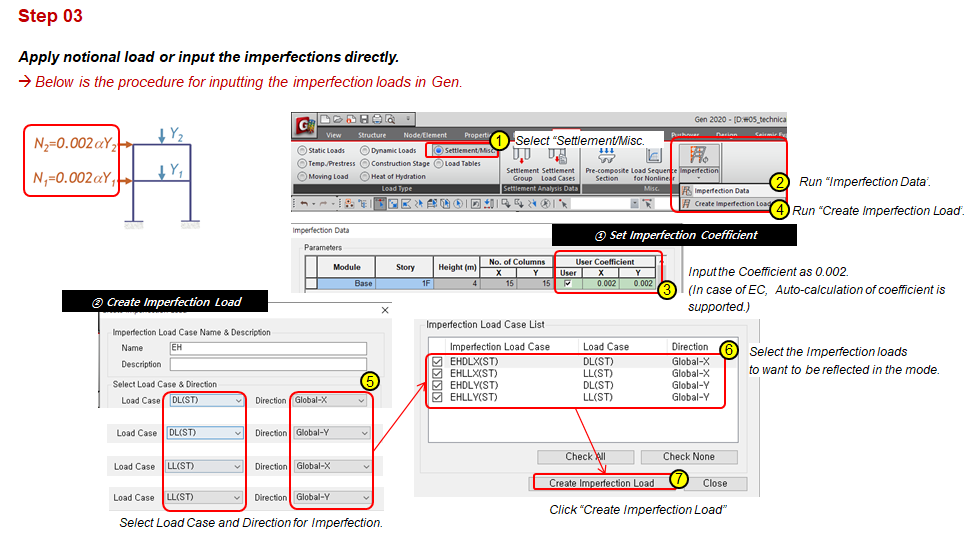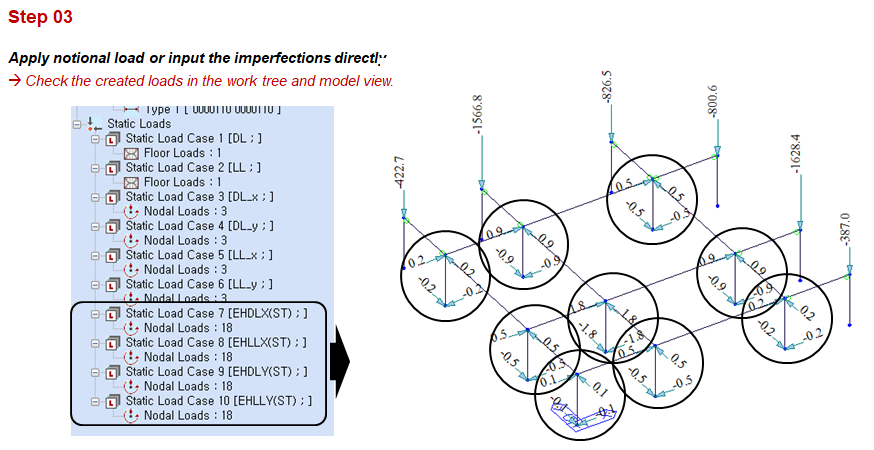Figure 8. The design procedure of direct analysis in Midas Gen (Step 3)

4) Step 4. Conduct a second-order analysis (“Rigorous” or B1-B2 amplification on first-order).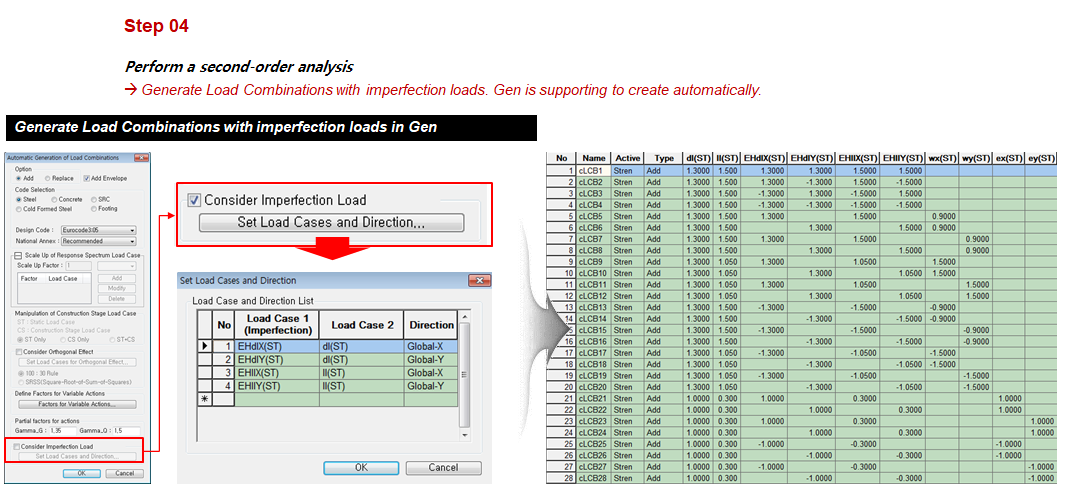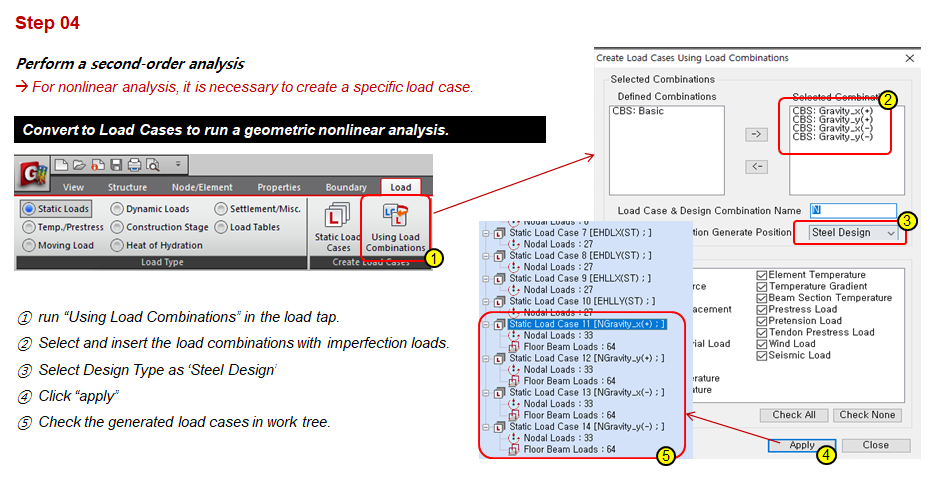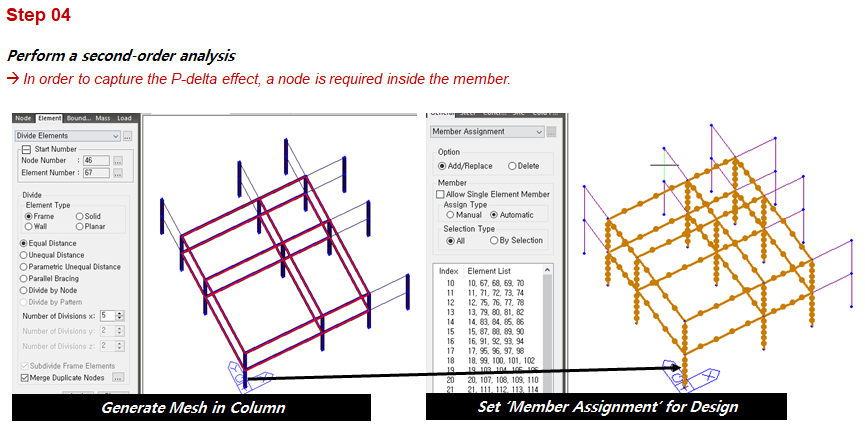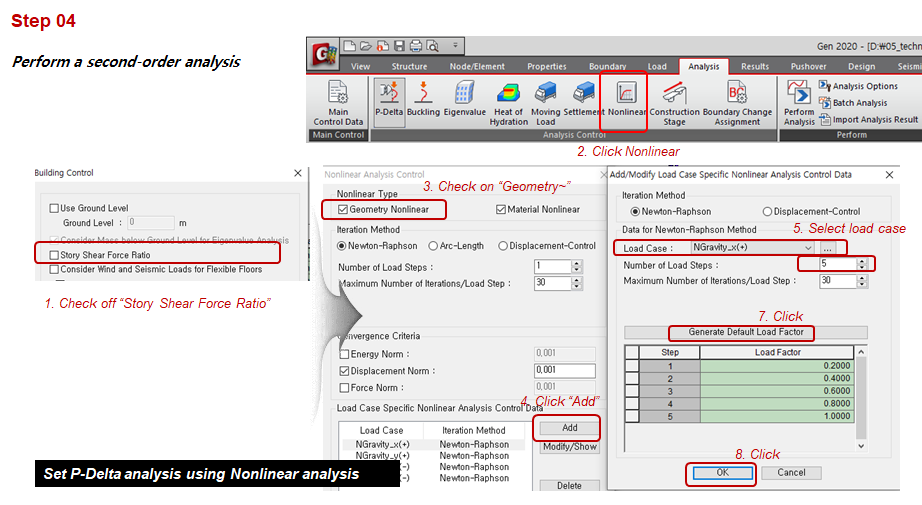Figure 9. The design procedure of direct analysis in Midas Gen (Step 4)

5) Step 5. Design members using AISC specification and K=1.0.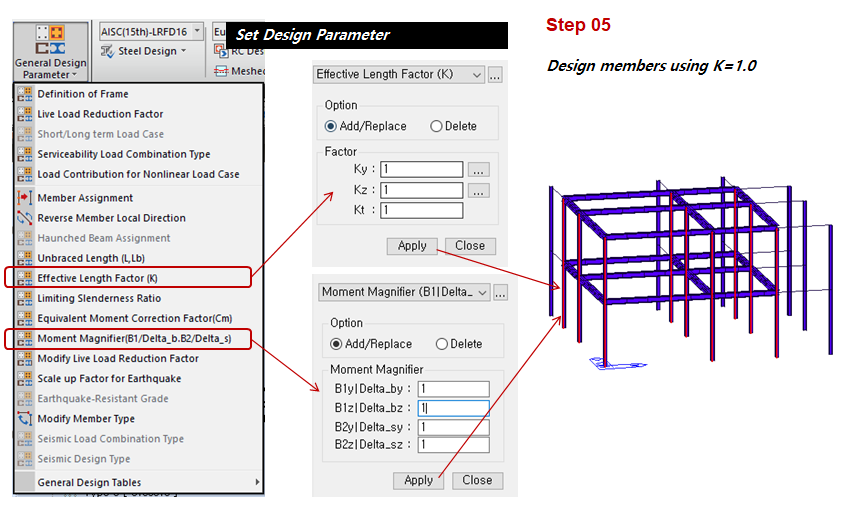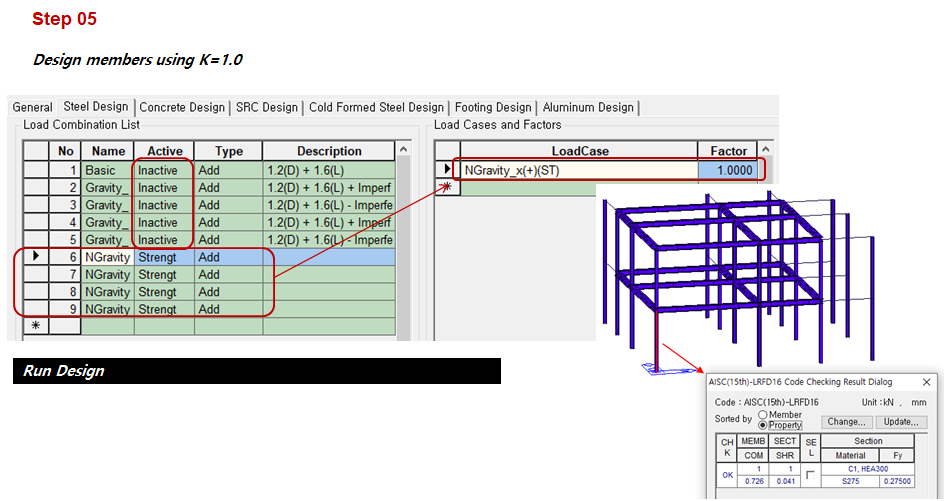Figure 10. The design procedure of direct analysis in Midas Gen (Step 5)

Author InformationYeong-il Seo | Principal Structural Engineer

Young-il has over 13+ years of experience in building design, especially high-rise buildings with column reduction analysis, plant structures, pushover analysis, health monitoring, and vibration control projects. Since 2016, he is planning and providing technical supports for midas building products such as midas Gen, nGen, and Design+.